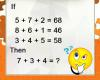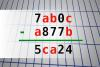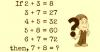BRAIN TEASERSBrain Teasers User Profile

# ASHANAND BAIRWA

rank
518
points
3
See full ranking list
short ranking list
 516 Ieva Kumpyte 3 517 amira msilini 3 518 ASHANAND BAIRWA 3 519 Faisal Nadeem 3 520 Hamey Idris 3
 Calculate 7+3+4 If 5+7+2=68, 8+6+1=48 and 3+4+5=58 then 7+3+4=?Find number abc If 7ab0c - a877b = 5ca24 find number abc. Multiple solutions may exist.Solve Math Puzzle If 2 + 3 = 8, 3 + 7 = 27, 4 + 5 = 32, 5 + 8 = 60, 6 + 7 = 72, then, 7 + 8 = ?